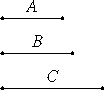# Proposition 19

Given three numbers, to investigate when it is possible to find a fourth proportional to them.Let A, B, and C be the given three numbers. It is required to investigate when it is possible to find a fourth proportional to them. [The Greek text of this Proposition is corrupt. However, analogously to Proposition 18 the condition that a fourth proportional to A, B, and C exists is that A measure the product of B and C. ]

Q.E.D.

## Guide

Note that a fourth proportional d to a, b and c has to satisfy a : b = c : d, so d would have to be bc/a. So the third proportional exists when a divides bc. No doubt that is what Euclid concludes in the missing part of this proposition.